# Figure Matrix - Non Verbal Reasoning questions

## Figure Matrix - Non Verbal Reasoning questions

In each of the following questions, find out which of the answer figures (1), (2), (3) and (4) completes the figure matrix ?

1. Select a suitable figure from the four alternatives that would complete the figure matrix.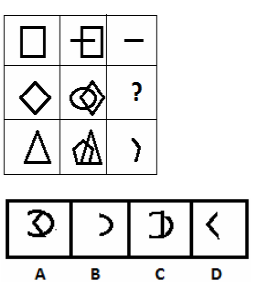i. A
ii. B
iii. C
iv. D

2. Select a suitable figure from the four alternatives that would complete the figure matrix.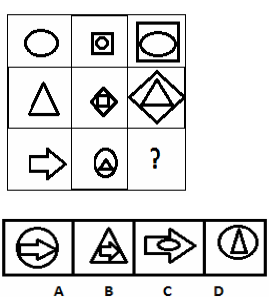i. A
ii. B
iii. C
iv. D

3. Select a suitable figure from the four alternatives that would complete the figure matrix.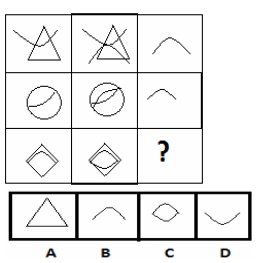i. A
ii. B
iii. C
iv. D

4. Select a suitable figure from the four alternatives that would complete the figure matrix.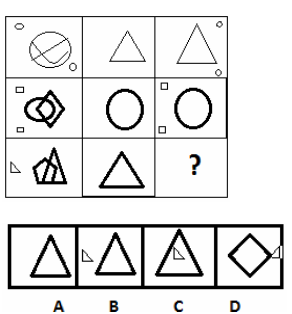i. B
ii. A
iii. D
iv. C

5. Select a suitable figure from the four alternatives that would complete the figure matrix.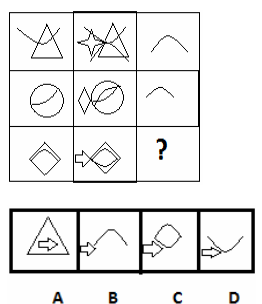i. A
ii. B
iii. C
iv. D

6. Select a suitable figure from the four alternatives that would complete the figure matrix.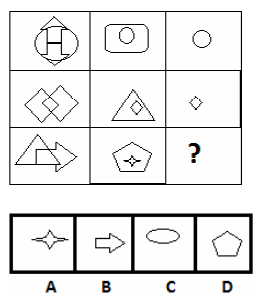i. B
ii. A
iii. C
iv. D

7. Select a suitable figure from the four alternatives that would complete the figure matrix.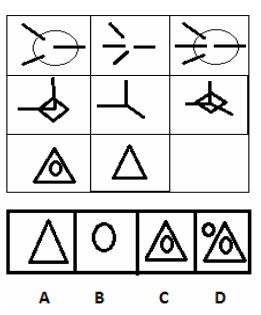i. A
ii. B
iii. C
iv. D

8. Select a suitable figure from the four alternatives that would complete the figure matrix.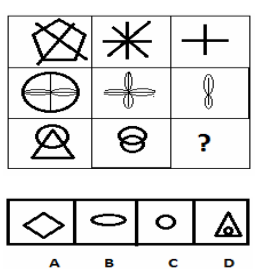i. A
ii. B
iii. C
iv. D

9. Select a suitable figure from the four alternatives that would complete the figure matrix.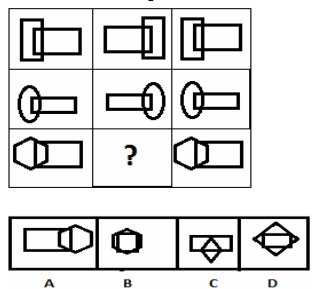i. A
ii. B
iii. C
iv. D

10. Select a suitable figure from the four alternatives that would complete the figure matrix.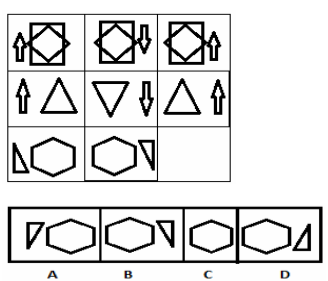i. B
ii. C
iii. A
iv. D

Q11. Select a suitable figure from the four alternatives that would complete the figure matrix.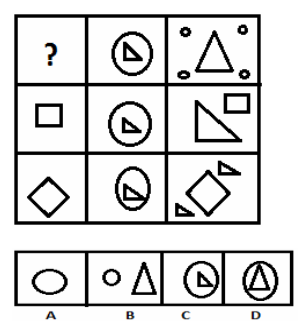i. A
ii. B
iii. C
iv. D

Q12. Select a suitable figure from the four alternatives that would complete the figure matrix.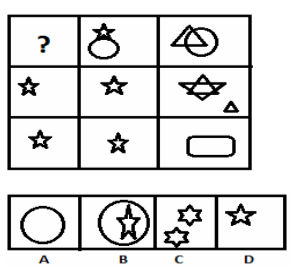i. B
ii. A
iii. C
iv. D

Q13. Select a suitable figure from the four alternatives that would complete the figure matrix.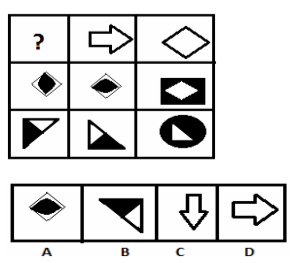i. A
ii. B
iii. C
iv. D

Q14. Select a suitable figure from the four alternatives that would complete the figure matrix.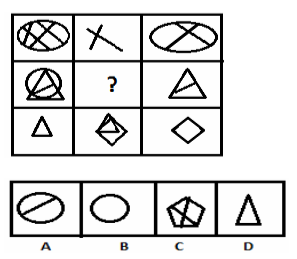i. A
ii. B
iii. D
iv. C

Q15. Select a suitable figure from the four alternatives that would complete the figure matrix.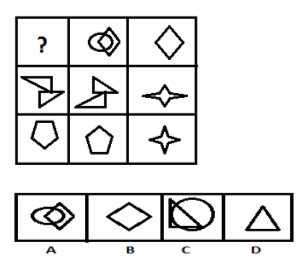i. A
ii. B
iii. C
iv. D

Q16. Select a suitable figure from the four alternatives that would complete the figure matrix.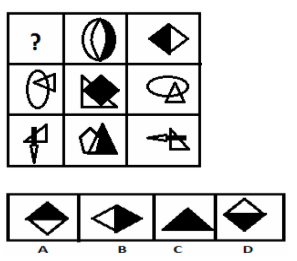i. A
ii. B
iii. C
iv. D

Q17. Select a suitable figure from the four alternatives that would complete the figure matrix.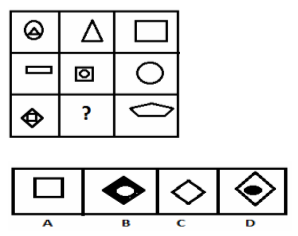i. D
ii. B
iii. A
iv. C

Q18. Select a suitable figure from the four alternatives that would complete the figure matrix.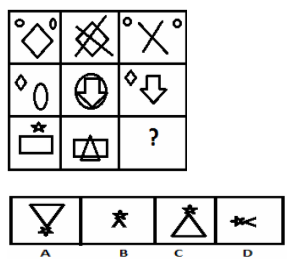i. A
ii. B
iii. C
iv. D

Q19. . Select a suitable figure from the four alternatives that would complete the figure matrix.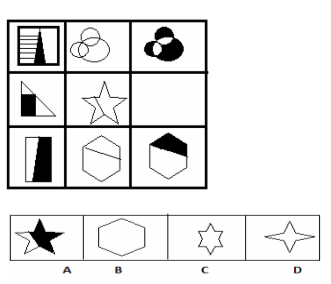i. A
ii. B
iii. C
iv. D

Q20. Select a suitable figure from the four alternatives that would complete the figure matrix.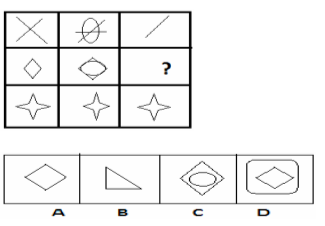i. A
ii. B
iii. C
iv. D

#### Discussion

• RE: Figure Matrix - Non Verbal Reasoning questions -gh (03/12/19)
• Answer for the third question is wrong
• RE: Figure Matrix - Non Verbal Reasoning questions -abcd (10/11/18)
• Qno.6 is wrong
• RE: Figure Matrix - Non Verbal Reasoning questions -tom (05/25/16)
• can you pls let me know the explaination for each question?.thanks
• RE: Figure Matrix - Non Verbal Reasoning questions -mani dhot (11/01/15)
• i get 20 out of 20
i really enjoy to solve these questions.
• RE: Figure Matrix - Non Verbal Reasoning questions -deepak (11/18/14)
• q6 is rong ans so try to again right way
• RE: Figure Matrix - Non Verbal Reasoning questions -Jason (09/02/14)
• As I age, I become dumber and dumber. A meagerly six questions I didn't understand.

Feel like a dunce!
• RE: Figure Matrix - Non Verbal Reasoning questions -Om Das (05/31/14)
• Hello,my name is Om Das I just turned 7 years old I am getting ready for my IQ test I found this website.It's pretty challenging and I liked it.Thank You